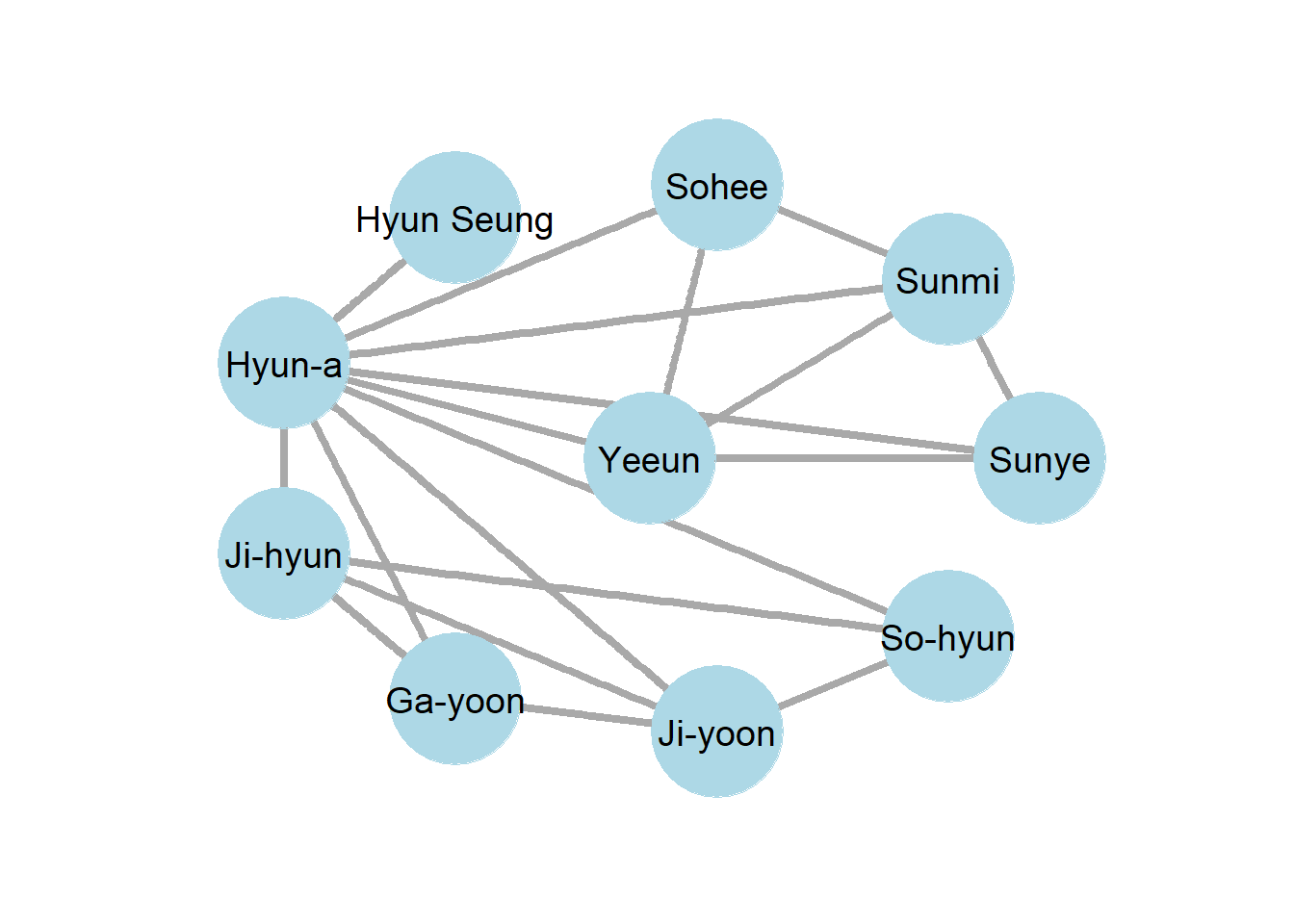## 2.14 Practice Problems

### 2.14.1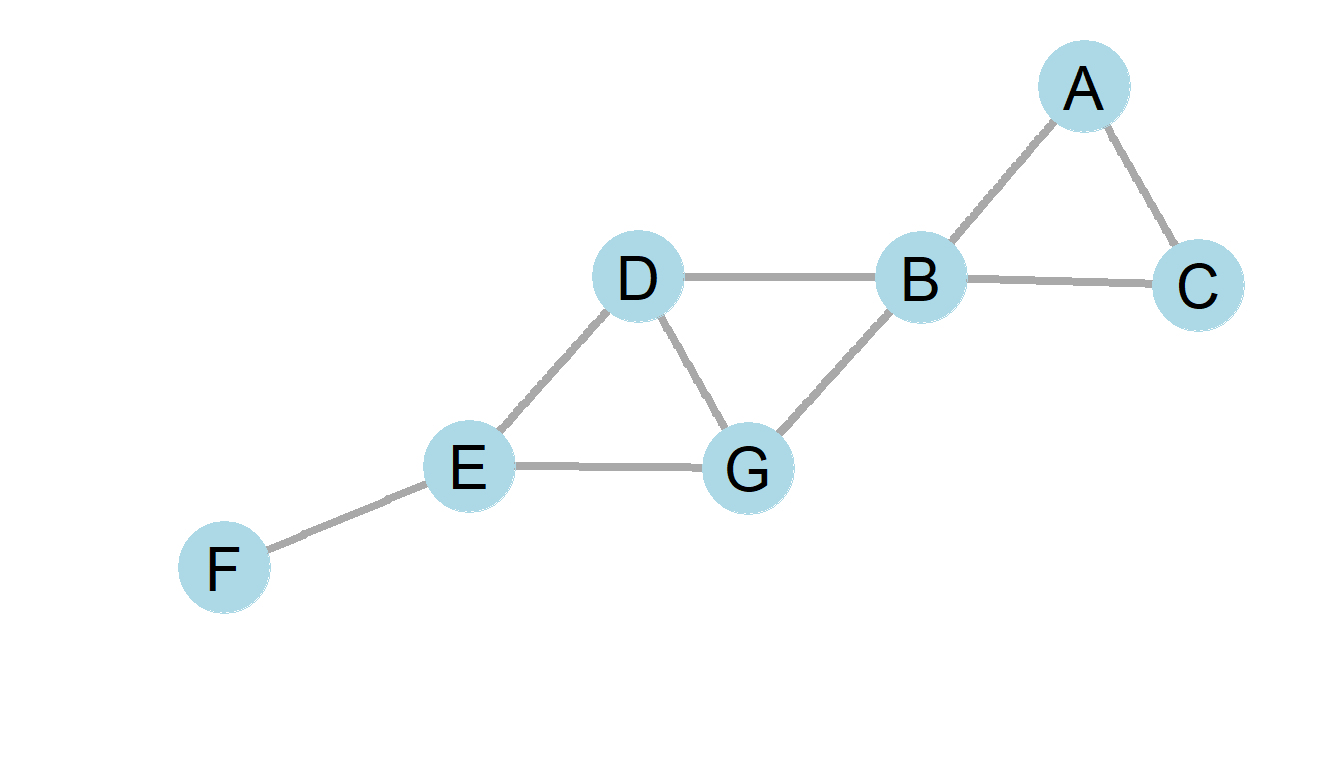1. What type of graph is pictured above? What type of relationships might the graph represent?
2. How many nodes and edges are in the above graph?
3. What is the average degree of the above graph?
4. What is modal degree in the above graph?
5. What is the degree distribution of the above graph?
6. What is the density of the above graph?

### 2.14.2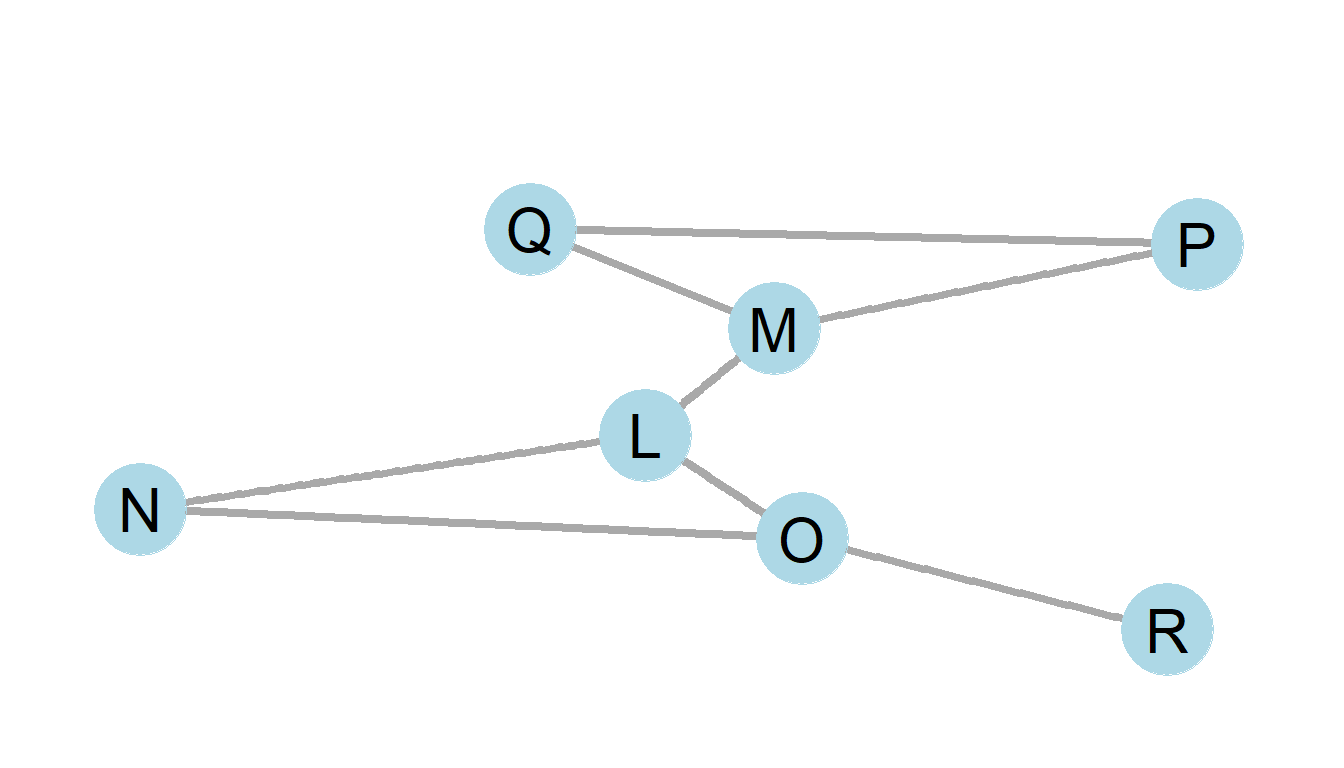1. What type of graph is pictured above? What type of relationships might the graph represent?
2. How many nodes and edges are in the above graph?
3. What is the average in-degree of the above graph? The average out-degree?
4. What is the density of the above graph?

### 2.14.3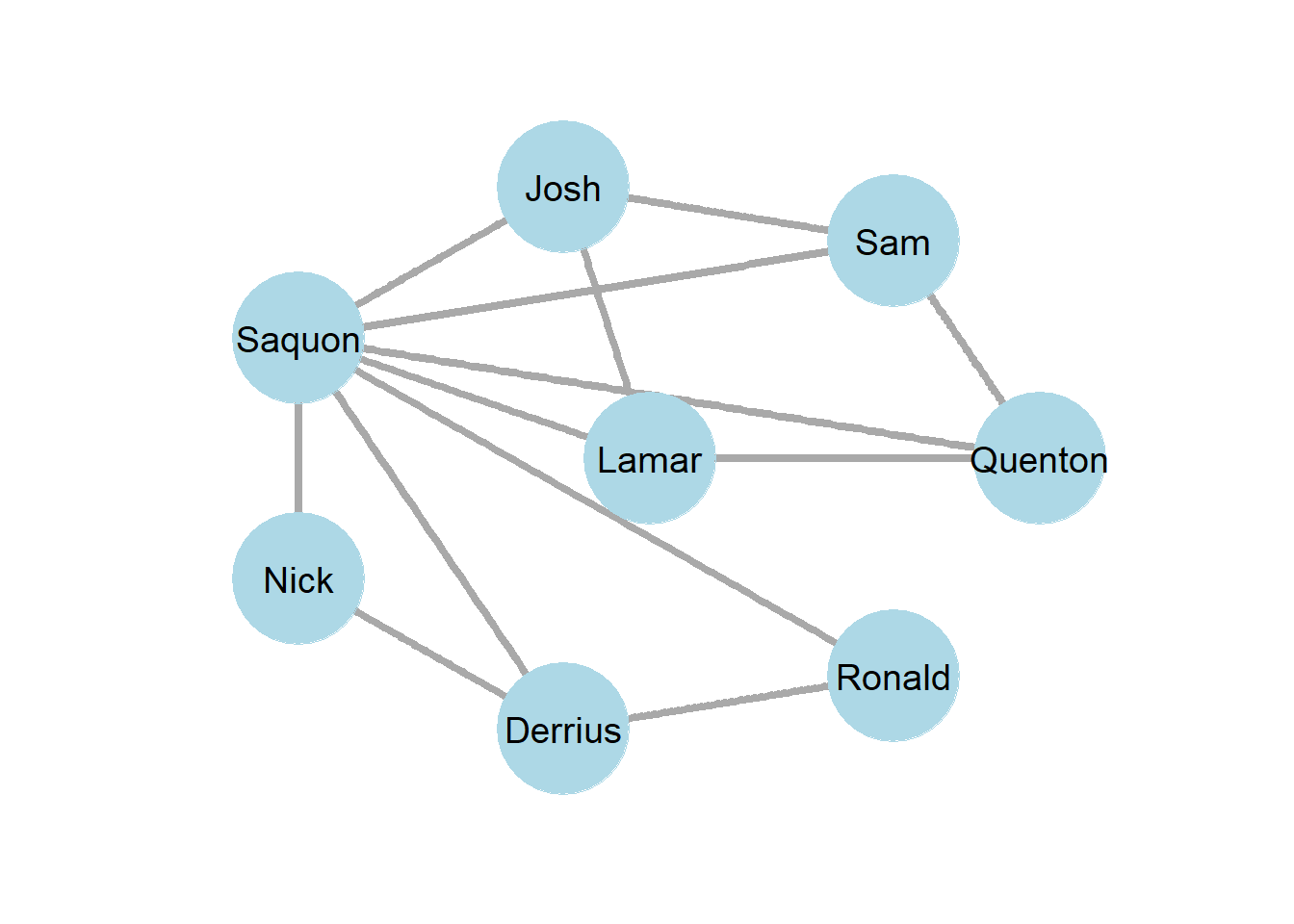### 2.14.4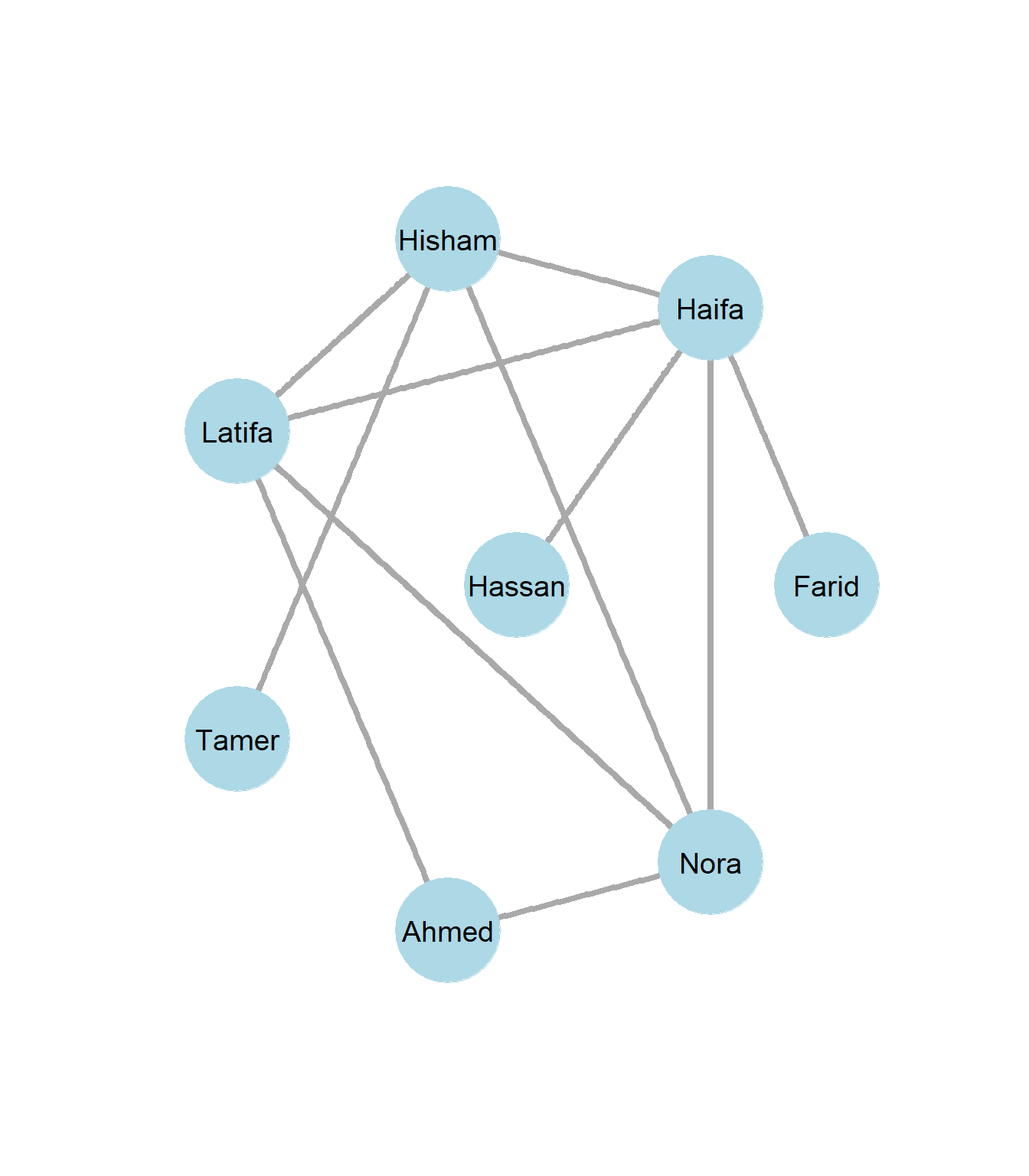### 2.14.5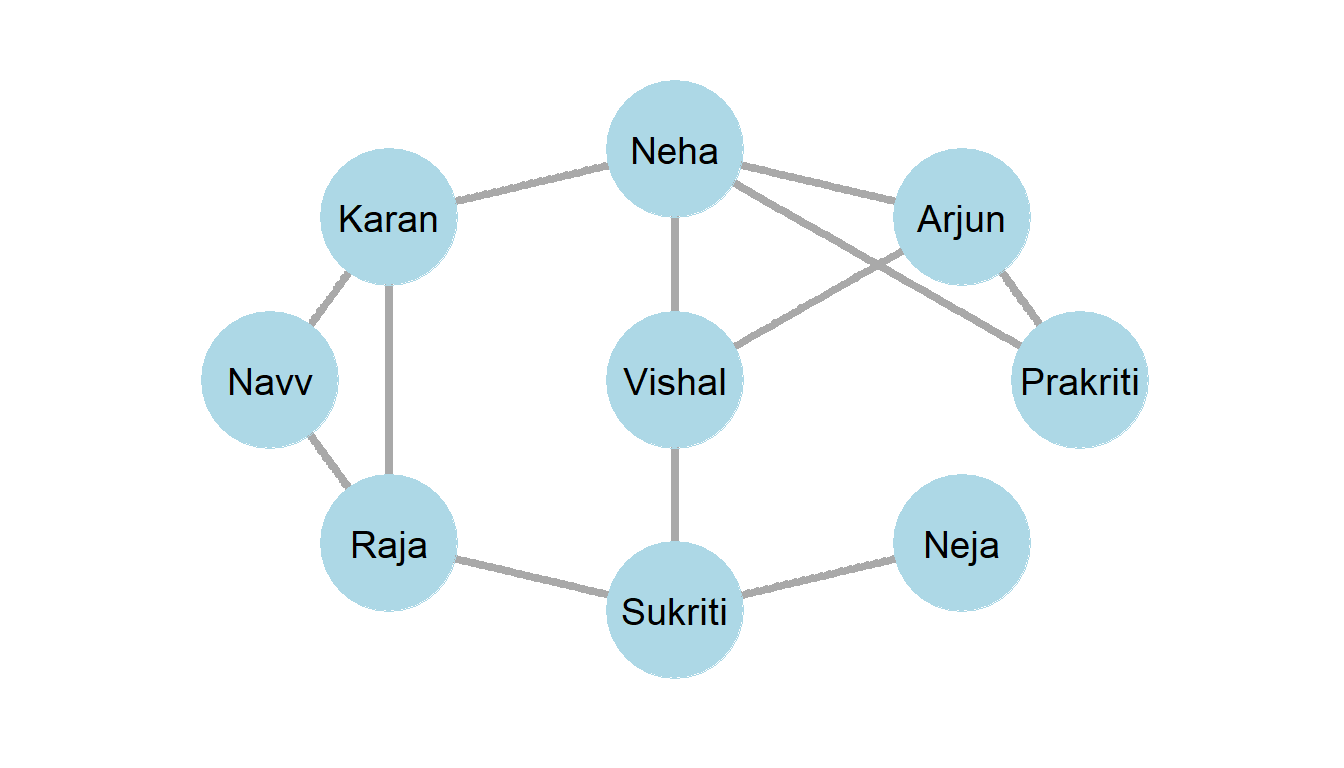### 2.14.6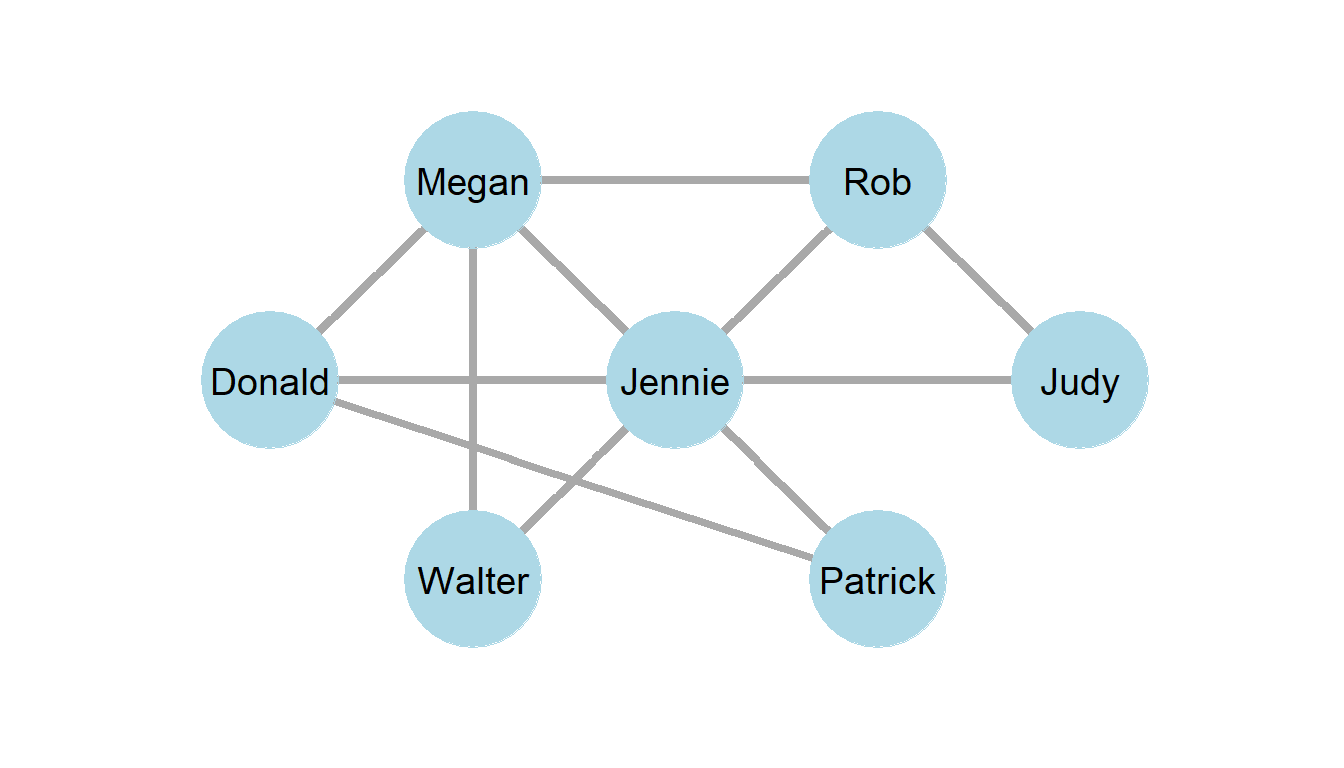1. Above is a graph of Jennie’s Ego Network. What is the size of the graph and the clustering coefficient/ego density?